# Finding a cycle in an undirected graph using Disjoint Sets

In this article, we are going to see how we can use disjoint set ADT to find whether there is a cycle in the undirected graph or not?
Submitted by Radib Kar, on September 11, 2020

Prerequisite:

In the previous article, we saw how we can find a cycle in an undirected graph using DFS? Here we are going to see how we can use disjoint set ADT operation to find whether there is a cycle or not efficiently. If you haven't gone through our articles on disjoint set ADT, please go through the prerequisite articles first to understand this discussion.

In the below example, graph 1 has a cycle where graph2 don't have any cycle.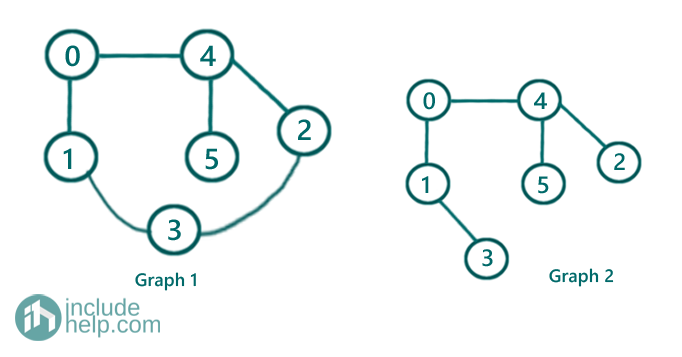So, the thing is how we can use disjoint set ADT to find whether there is a cycle or not. As we have discussed in the pre-requisite articles, that an edge is a relation b/w two nodes and two nodes having an edge b/w them, are supposed to be in the same disjoint set. So through our ADT operation FIND(X) & UNION(X, Y), we create disjoint sets out of the available edges. So, to detect a cycle in an undirected graph, we can use the same idea. The algorithm would be:

For each edge in the edge list:

Find parents(set name) of the source and destination nodes respectively (Though we are using terms like source & destination node, the edges are undirected).

If both have the same parent, that means they belong to the same set already and this edge creates a cycle. Since both the node already belong to the same set, that means even without this edge we could have reached one node from other. So the addition of this edge creates a cycle since edges are undirected.

Else

Do the UNION of them to bring them under the same set.

That's so simple!! But only if you have the idea of disjoint set ADT.

Below is the implemenatation.

```#include <bits/stdc++.h>
using namespace std;

int find(int x, vector<int>& parent)
{
if (parent[x] == x)
return x;
return find(parent[x], parent);
}

void UNION(int x, int y, vector<int>& parent, vector<int>& rank)
{
if (rank[x] > rank[y]) {
parent[y] = x;
rank[x]++;
}
else if (rank[x] < rank[y]) {
parent[x] = y;
rank[y]++;
}
else
parent[y] = x;
rank[x]++;
}

bool isCyclic(vector<vector<int> >& edges, int V)
{
vector<int> parent(V);

for (int i = 0; i < V; i++)
parent[i] = i;
vector<int> rank(V, 0);

for (auto edge : edges) {
int x = find(edge, parent);
int y = find(edge, parent);
if (x != y)
UNION(x, y, parent, rank);
else
return true;
}
return false;
}

int main()
{
int n, m;

cout << "Enter the number of edges\n";
cin >> n;
cout << "Enter the number of nodes\n";
cin >> m;

//edges list
//each edge is a vector [u,v]
vector<vector<int> > edges(n);

for (int i = 0; i < n; i++) {
int u, v;
cout << "info for edge " << i + 1 << endl;
cout << "Enter source node u: ";
cin >> u;
cout << "Enter destination node v: ";
cin >> v;
edges[i] = vector<int>{ u, v };
}

if (isCyclic(edges, m))
cout << "There is a cycle in the graph\n";
else
cout << "There is no cycle in the graph\n";

return 0;
}
```

Output:

```RUN 1:
Enter the number of edges
6
Enter the number of nodes
6
info for edge 1
Enter source node u: 0
Enter destination node v: 1
info for edge 2
Enter source node u: 0
Enter destination node v: 4
info for edge 3
Enter source node u: 1
Enter destination node v: 3
info for edge 4
Enter source node u: 4
5Enter destination node v: 5
info for edge 5
Enter source node u: 4
Enter destination node v: 2
info for edge 6
Enter source node u: 2
Enter destination node v: 3
There is a cycle in the graph

RUN 2:
Enter the number of edges
5
Enter the number of nodes
6
info for edge 1
Enter source node u: 0
Enter destination node v: 1
info for edge 2
Enter source node u: 1
Enter destination node v: 3
info for edge 3
Enter source node u: 0
Enter destination node v: 4
info for edge 4
Enter source node u: 4
Enter destination node v: 5
info for edge 5
Enter source node u: 4
Enter destination node v: 2
There is no cycle in the graph
```

In the above implementation, we see a more efficient implementation of union where we have used "rank of the set". Rank is another array like parent array where we store the height of the tree (which is the root for the set). The motive behind using rank is to balance the heights, otherwise, the complexity for FIND(X) will increase.

Dry Run:

So the edge list is:

[ [0, 1],

[0, 4],

[1, 3],

[4, 5],

[4, 2],

[2, 3] ]

We will process each edge one by one and do necessary FIND & UNION

Initially, parent[] is: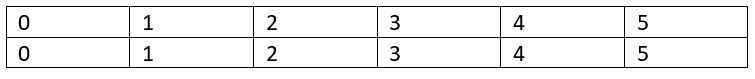Edge 1:

So the source is 0 and destination is 1

We will find the parent of both 0 & 1

Finding parent of 0

parent is 0 so it returns 0

Finding parent of 1

parent is 1 so it returns 1

Since both their set names( parent) are different we do a union

I am skipping the rank part( you can do that your own)

Thus parent=0 now, so after processing edge 1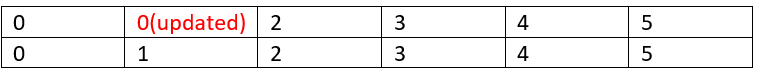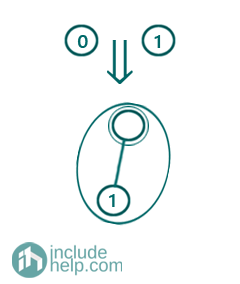Edge 2:

So the source is 0 and destination is 4

We will find the parent of both 0 & 4

Finding parent of 0

parent is 0 so it returns 0

Finding parent of 4

parent is 4 so it returns 4

Since both their set names( parent) are different we do a union

Thus parent=0 now, so after processing edge 2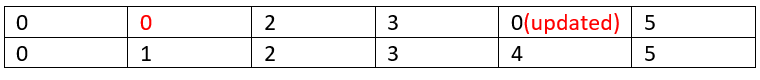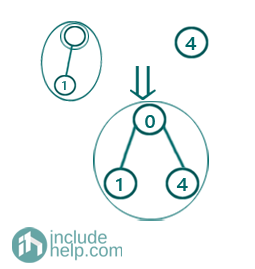Edge 3:

So the source is 1 and destination is 3

We will find the parent of both 1 & 3

Finding parent of 1

parent is 0 so it returns find(0, parent) and that returns 0 ultimately (parent== 0)

Finding parent of 3

parent is 3 so it returns 3

Since both their set names( parent) are different we do a union

Thus parent=0 now, so after processing edge 3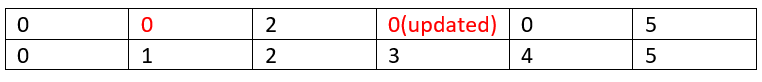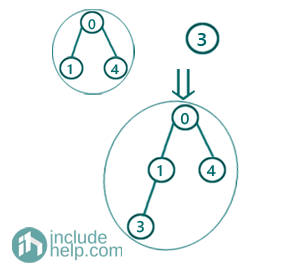Edge 4:

So the source is 4 and destination is 5

We will find the parent of both 4 & 5

Finding parent of 4

parent is 0 so it returns find(0, parent) and that returns 0 ultimately (parent==0)

Finding parent of 5

parent is 5 so it returns 5

Since both their set names( parent) are different we do a union

Thus parent=0 now, so after processing edge 4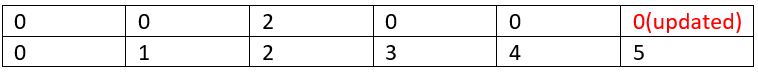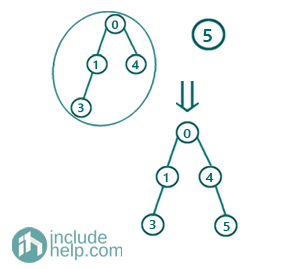Edge 5:

So the source is 4 and destination is 2

We will find the parent of both 4 & 2

Finding parent of 4

parent is 0 so it returns 0

Finding parent of 2

parent is 2 so it returns 2

Since both their set names( parent) are different we do a union

Thus parent=0 now, so after processing edge 5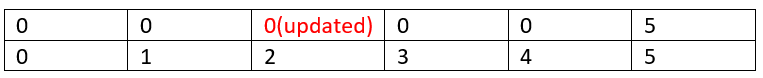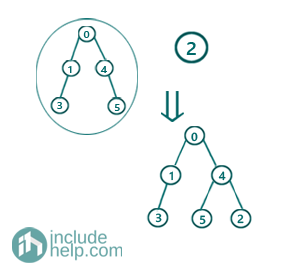Edge 6:

So the source is 2 and destination is 3

We will find the parent of both 2 & 3

Finding parent of 2

parent is 0 so it returns find(0, parents) which ultimately returns 0

Finding parent of 3

parent is 0 and thus it returns 0

So, that means both of the nodes already belongs to the same set, that means this edge result in a cycle.

Languages: » C » C++ » C++ STL » Java » Data Structure » C#.Net » Android » Kotlin » SQL
Web Technologies: » PHP » Python » JavaScript » CSS » Ajax » Node.js » Web programming/HTML
Solved programs: » C » C++ » DS » Java » C#
Aptitude que. & ans.: » C » C++ » Java » DBMS
Interview que. & ans.: » C » Embedded C » Java » SEO » HR
CS Subjects: » CS Basics » O.S. » Networks » DBMS » Embedded Systems » Cloud Computing
» Machine learning » CS Organizations » Linux » DOS
More: » Articles » Puzzles » News/Updates